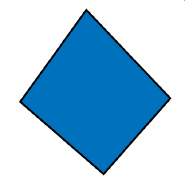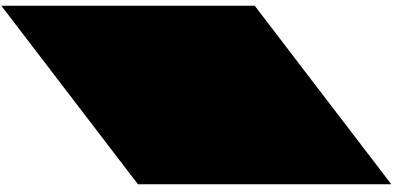5.G.B.3 - 2 dimensional shapes
 Name:    5.G.B.3 - 2 dimensional shapes

True/False
Indicate whether the statement is true or false.

1.

The shape below is a paralleogram, TRUE or FALSE?2.

The shape below is a square, TRUE or FALSE?3.

The shape below is a trapezoid, TRUE or FALSE?4.

The shape below is a parallelogram, TRUE or FALSE?5.

The shape below is a square, TRUE or FALSE?Multiple Response
Identify one or more choices that best complete the statement or answer the question.

6.

Click all the words that describe the shape below.a. rhombus c. quadrilateral b. parallelogram d. square

7.

Click all the words that describe the shape below.a. rhombus c. quadrilateral b. parallelogram d. square

8.

Click all the words that describe the object below.a. parallelogram c. trapezoid b. quadrilateral d. rhombus

9.

Click all the words that describe the shape below.a. square c. rectangle b. trapezoid d. quadrilateral

10.

Click all the words that describe the shape below.a. rectangle c. parallelogram b. rhombus d. quadrilateral